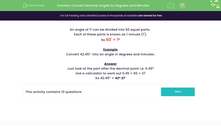# Convert Decimal Angles to Degrees and Minutes

In this worksheet, students revise conversion of angles given in decimal format to angles in degrees and minutes.Key stage:  KS 3

Curriculum topic:   Number

Curriculum subtopic:   Use Standard Units of Measure

Difficulty level:#### Worksheet Overview

An angle of 1º can be divided into 60 equal parts.

Each of these parts is known as 1 minute (1').

So 60' = 1º

Example

Convert 42.45º  into an angle in degrees and minutes.

Just look at the part after the decimal point i.e. 0.45º

Use a calculator to work out 0.45 × 60 = 27

So 42.45º = 42º 27'

### What is EdPlace?

We're your National Curriculum aligned online education content provider helping each child succeed in English, maths and science from year 1 to GCSE. With an EdPlace account you’ll be able to track and measure progress, helping each child achieve their best. We build confidence and attainment by personalising each child’s learning at a level that suits them.

Get started